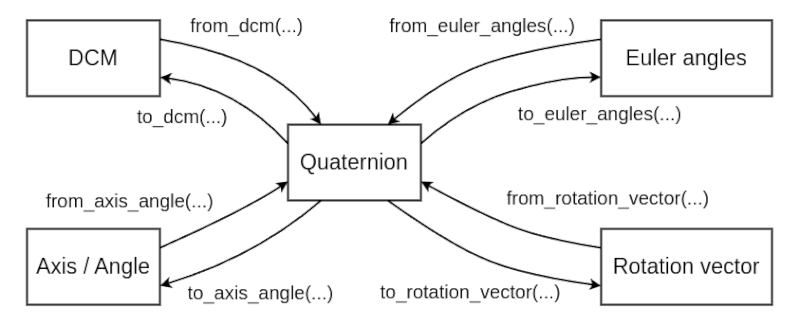## no-std quaternion-core

Provides quaternion operations and interconversion with several attitude representations

### 10 releases

 0.4.2 Jun 2, 2023 May 28, 2023 Feb 4, 2023 Dec 25, 2022 May 7, 2022

#128 in Math

Used in quaternion-wrapper

MIT/Apache

82KB
1K SLoC

# quaternion-coreQuaternion library written in Rust.

This provides Quaternion operations and interconversion with several attitude representations as generic functions (supports `f32` & `f64`).

Additionally, it also works in a `no_std` environment!

## Usage

Add this to your `Cargo.toml`:

``````[dependencies]
quaternion-core = "0.4"
``````

For use in a `no_std` environment:

``````[dependencies.quaternion-core]
version = "0.4"
default-features = false
features = ["libm"]
``````

## ConversionInterconversion with 24 different euler angles (12 each of `Intrinsic` and `Extrinsic`) is possible!!

Other interconversions with axis/angle and rotation vector are also possible.

## Features

### fma

When this feature is enabled, the mul_add method will be used internally as much as possible. That is, `(s * a) + b` will be expanded as `s.mul_add(a, b)` at compile time.

This crate uses the `mul_add` method mainly to improve calculation speed, but if the CPU does not support the `FMA` (Fused Multiply-Add) instruction or if the `libm` feature is enabled, then the calculation is performed by the software implementation. In this case, it may be rather slower than if the `fma` feature is not enabled.

### libm

If you set `default-features=false` (do not import `std`), you must enable this feature.

In this case, mathematical functions (e.g. `sin`, `cos`, `sqrt` ...) are provided by libm crate.

### norm-sqrt

When this feature is enabled, the default `norm(a)` implementation is compiled with `dot(a, a).sqrt()` instead.

By default, the `norm(a)` function is implemented in such a way that overflow and underflow are less likely to occur than with `dot(a, a).sqrt()`. However, if extremely large values are not input and underflow is not that much of a concern, `dot(a, a).sqrt()` is sufficient (and `dot(a, a).sqrt()` is faster than the default implementation in most cases).

## Example

`src/main.rs`:

``````use quaternion_core as quat;

const PI: f64 = std::f64::consts::PI;
const EPSILON: f64 = 1e-12;

fn main() {
// Generates a quaternion representing the
// rotation of π/2[rad] around the y-axis.
let q = quat::from_axis_angle([0.0, 1.0, 0.0], PI/2.0);

// Rotate the point.
let r = quat::point_rotation(q, [2.0, 2.0, 0.0]);

// Check if the calculation is correct.
let diff = quat::sub([0.0, 2.0, -2.0], r);
for val in diff {
assert!( val.abs() < EPSILON );
}
}
``````

## Releases

Release notes are available in RELEASES.md.